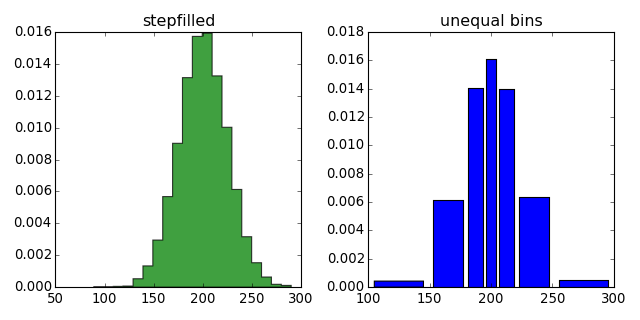Previous topic

statistics example code: histogram_demo_features.py

Next topic

statistics example code: histogram_demo_multihist.py

statistics example code: histogram_demo_histtypes.py¶```"""
Demo of the histogram (hist) function with different ``histtype`` settings.

* Histogram with step curve that has a color fill.
* Histogram with with unequal bin widths.

"""
import numpy as np
import matplotlib.pyplot as plt

mu = 200
sigma = 25
x = mu + sigma*np.random.randn(10000)

fig, (ax0, ax1) = plt.subplots(ncols=2, figsize=(8, 4))

ax0.hist(x, 20, normed=1, histtype='stepfilled', facecolor='g', alpha=0.75)
ax0.set_title('stepfilled')

# Create a histogram by providing the bin edges (unequally spaced).
bins = [100, 150, 180, 195, 205, 220, 250, 300]
ax1.hist(x, bins, normed=1, histtype='bar', rwidth=0.8)
ax1.set_title('unequal bins')

plt.tight_layout()
plt.show()
```

Keywords: python, matplotlib, pylab, example, codex (see Search examples)